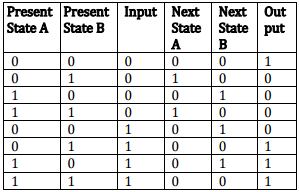# CS GATE 2009 - Online Test

Q1. What is the chromatic number of an n-vertex simple connected graph which does not contain any odd length cycle? Assume n ≥ 2
Explaination / Solution:
No Explaination.

Q2. Which one of the following is TRUE for any simple connected undirected graph with more than 2 vertices?
Explaination / Solution:
No Explaination.

Q3. What is the minimum number of gates required to implement the Boolean function (AB + C) if we have to use only 2-input NOR gates?
Explaination / Solution:
No Explaination.

Q4. How many 32K × 1 RAM chips are needed to provide a memory capacity of 256 K-bytes =
Explaination / Solution:
No Explaination.

Q5. In which one of the following page replacement policies, Belady’s anomaly may occur?
Explaination / Solution:
No Explaination.

Q6. S → a Sa| bSb| a| b The language generated by the above grammar over the alphabet {a, b} is the set of
Explaination / Solution:
No Explaination.

Q7. Let πbe a problem that belongs to the class NP. Then which one of the following is TRUE?
Explaination / Solution:
No Explaination.

Q8. Given the following state table of an FSM with two states A and B, one input and one output:If the initial state is A = 0, B = 0, what is the minimum length of an input string which will take the machine to the state A = 0, B = 1 with Output = 1?
Explaination / Solution:
No Explaination.

Q9. A CPU generally handles an interrupt by executing an interrupt service routine
Explaination / Solution:
No Explaination.

Q10. What is the number of swaps required to sort n elements using selection sort, in the worst case?
Explaination / Solution:
No Explaination.